Browse by Topic
Related Topics

# From Close to Perfect—A Triangle Problem

RootApproximant can turn an approximate solution into a perfect solution, such as for a square divided into fifty 45°-60°-75° triangles.

A square can be divided into triangles, for example by connecting opposite corners. It’s possible to divide a square into seven similar but differently sized triangles or ten acute isosceles triangles. Classic puzzles involve cutting a square into eight acute triangles, or twenty 1 – 2 – √5 triangles. The last image uses 45°-60°-75° triangles, but one triangle has a flaw.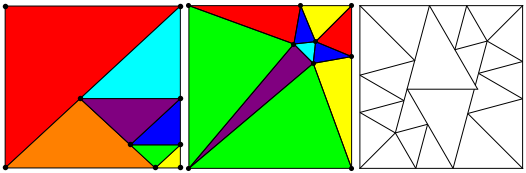It’s easy to divide a square with similar right triangles. Can a square be divided into similar non-right triangles? In his paper “Tilings of Polygons with Similar Triangles” (Combinatorica, 10(3), 1990 pp. 281–306), Laczkovich proved exactly three triangles provided solutions, with angles 22.5°-45°-122.5°, 15°-45°-120°, and 45°-60°-75°. I read his paper to try to make an image for the 45°-60°-75° case, but his construction was complex, and seemed to require thousands of triangles, so I tried to find my own solutions. All my attempts had flaws, such as the last image above, so I made a contest out of it: \$200, minus a dollar for every triangle in the solution.

Lew Baxter started with a close examination of the Laczkovich solution, and got it to work with 7 trillion triangles. But he saw ways to improve it, and wrote a program to search for improvements. Within several weeks, he had a 198 triangle solution, enough to win \$2 from me. He kept at it, and finally came up with a 64 triangle solution. Unfortunately, all the points were approximate. It was possible that the solution he sent, shown here, might have been close, but not perfect: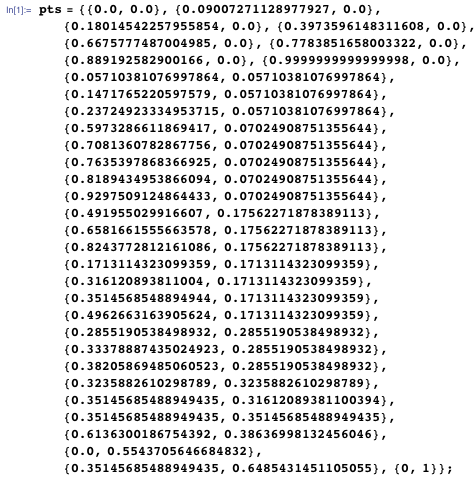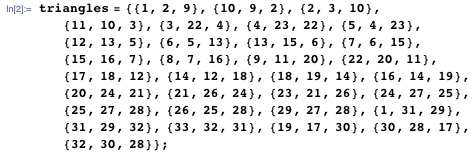Making the solution perfect looked like a job for RootApproximant, which finds a root of a polynomial that is close to a desired value. In geometric constructions such as these, it’s not unlikely that the exact values are indeed polynomial roots. For the problem in question, I tried the following. Multiplying by 4686 eliminated a lot of fractions. All the values were of the form a + b √3, which seemed very promising.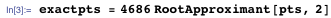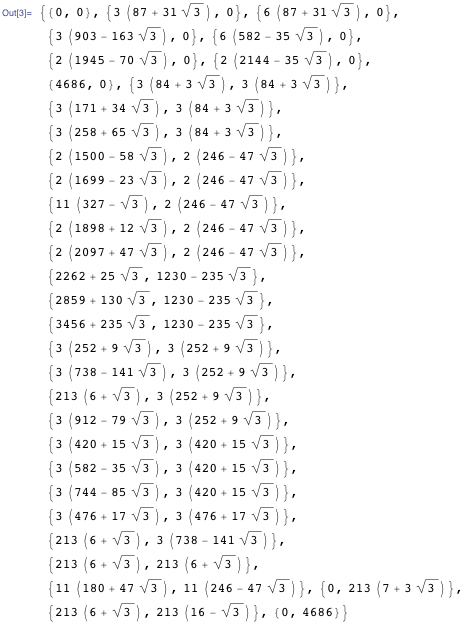We can then take a look at the proposed 64 triangle solution.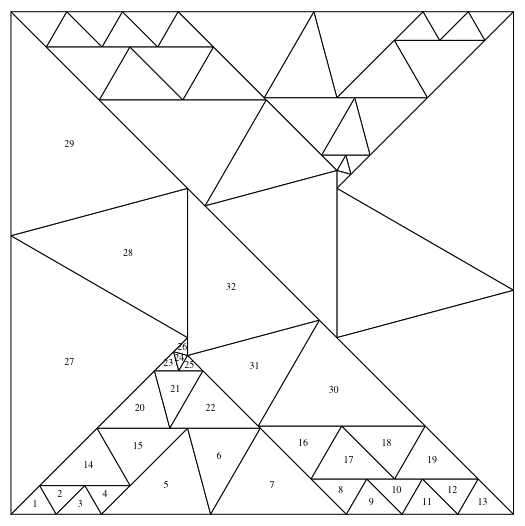Then I got a new message from Lew—if triangles 6, 7, 21, and 22 are flipped, then eight of the triangles can be replaced by just one triangle.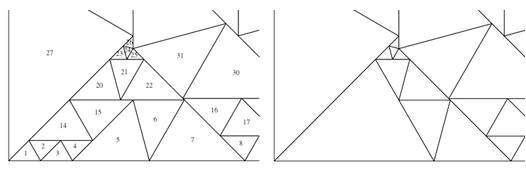It wasn’t quite that easy, but I calculated a new set of points.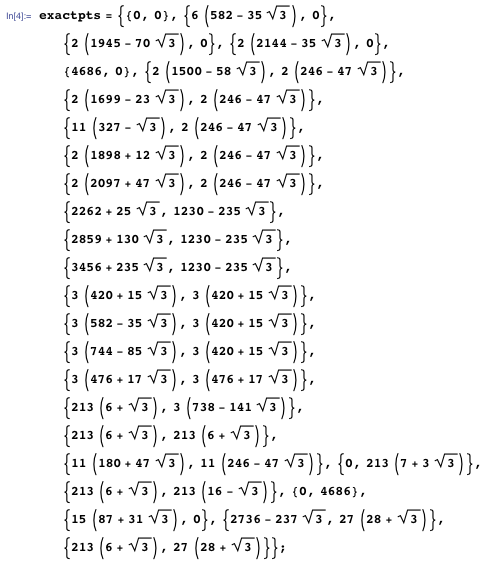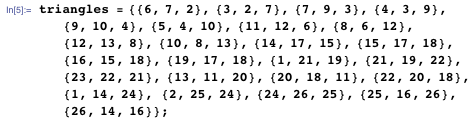That leads to a 50 triangle solution. So is that a perfect solution? With code for calculating an angle based on vertices, all the angles can be checked.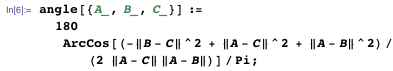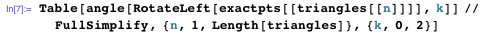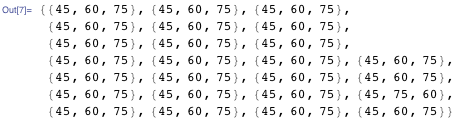All of the triangles are exact 45°-60°-75° triangles, so Baxter’s solution is indeed exact. Now we can take a look at the 50 triangle solution.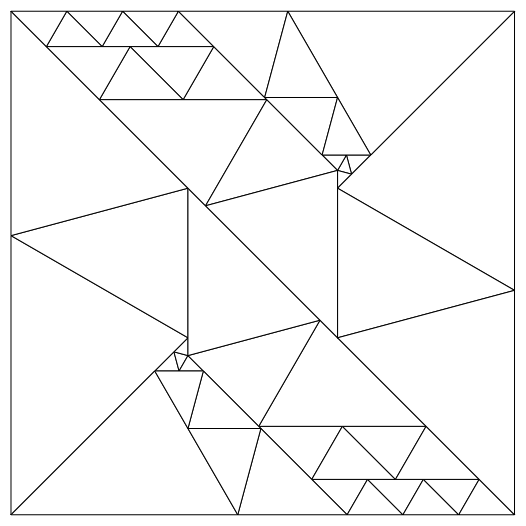So I owe Lew Baxter \$150. If you’re ever tackling problems in numerical optimization, and the values are mysterious, it’s worth giving RootApproximant a try. You might have an exact solution.